# Difference between revisions of "Catalan constant"

Named after its inventor, E.Ch. Catalan (1814–1894), the Catalan constant(which is denoted also by) is defined by(a1)If, in terms of the Digamma (or Psi) function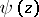, defined by(a2)

orone puts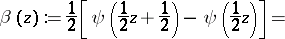(a3)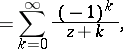where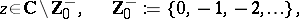then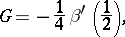(a4)

which provides a relationship between the Catalan constantand the Digamma function.

The Catalan constantis related also to other functions, such as the Clausen function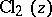, defined by(a5)and the Hurwitz zeta function, which is defined, when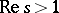, by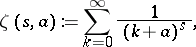(a6)Thus,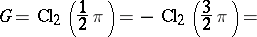(a7)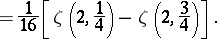Since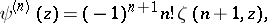(a8)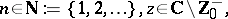the last expression in (a7) would follow also from (a4) in light of the definition in (a3).

A fairly large number of integrals and series can be evaluated in terms of the Catalan constant. For example,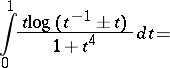(a9)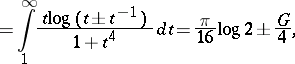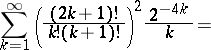(a10)and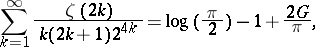(a11)

where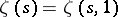denotes the familiar Riemann zeta-function.

How to Cite This Entry:
Catalan constant. Encyclopedia of Mathematics. URL: http://encyclopediaofmath.org/index.php?title=Catalan_constant&oldid=38979
This article was adapted from an original article by Hari M. Srivastava (originator), which appeared in Encyclopedia of Mathematics - ISBN 1402006098. See original article